Social QuestionCan you find a second relationship between 3 consecutive Fibonacci numbers?

Asked by LostInParadise (29303) December 19th, 2011
9 responses
“Great Question” (5)

The Fibonacci series has a long history in mathematics. It has a curious habit of turning up in various places in nature.

The series is defined very simply. The first two members are 1 and 1. Each subsequent member is defined as the sum of the previous two, so the first few terms are:
1 1 2 3 5 8 13 21 34.

Given 3 consecutive Fibonacci numbers, f(n), f(n+1) and f(n+2), the defining relationship between them is f(n+2) = f(n) + f(n+1). See if you can discover another relationship. The equation varies slightly, depending on whether n is even or odd.Follow this questionSend to a friend!Topics: , ,
Observing members: 0Composing members: 0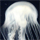I literally cannot visualize any of this.

whitetigress (3129)“Great Answer” (0)Do you mean that you do not see how the series is generated?
1+1 =2, giving 1 1 2
1+2 = 3 giving 1 1 2 3
2+3=5 1 1 2 3 5
and so on.

LostInParadise (29303)“Great Answer” (0)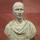1,2,3 are ordinal numbers.

FireMadeFlesh (16573)“Great Answer” (0)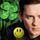I won’t give it away. But here’s a hint. .Take two neighboring numbers in the series and divide the first by the second, for example: ⅔= .666 3/5= .60 5/8= .625 8/13= .615 As you work your way to infinity you will see they converge on a truly golden number. You can work magic with two golden ratios next to each other.

LuckyGuy (40293)“Great Answer” (2)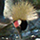Ratio between them converges to phi.

6rant6 (13697)“Great Answer” (0)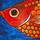f(n+2)*f(n) – f(n+1)*f(n+1) = (-1)exp(n)
That looks a bit clumsy, but if you multiply the largest by the smallest and take away the square of the middle number you will always get either +1 or -1.

flutherother (32181)“Great Answer” (3)That’s it!
For extra credit, you can do an inductive proof.

The formula can be derived from a more general result, which I learned about from the section on Fibonacci in Strange Curves, which is a really good popular math book.

LostInParadise (29303)“Great Answer” (3)Assume it is true for k then Fk+2Fk-F2k+1 = (-1)k
And Fk+2(Fk+2-Fk+1)-F2k+1= (-1)k
F2k+2 – (Fk+2.Fk+1)-F2k+1 = (-1)k
F2k+2—(Fk+2+Fk+1)Fk+1=(-1)k
F2k+2 –Fk+3Fk+1 =(-1)k
Reversing the signs Fk+3Fk+1-F2k+2 =(-1)k+1
Therefore it is true for k+1

flutherother (32181)“Great Answer” (1)Here is the way I did it.
Given that f(k+2)f(k) – f^2(k+1) = (-1)^k
We want to show that f(k+3)f(k+1) -f^2(k+2) = (-1)^(k+1)

f(k+3)f(k+1) -f^2(k+2) =
(f(k+2) + f(k+1))f(k+1) – f^2(k+1) =
f^2(k+1) + f(k+2)f(k+1) – f^2(k+1) = (using the inductive assumption)
f(k+2)f(k) – (-1)^k + f(k+2)f(k+1) – f^2(k+2) =
f(k+2)f(k) + (-1)^(k+1) + f(k+2)(f(k+1) – f(k+2)) =
f(k+2)f(k) + (-1)^(k+1) + f(k+2)(-f(k)) =
-1(k+1)

LostInParadise (29303)“Great Answer” (1)